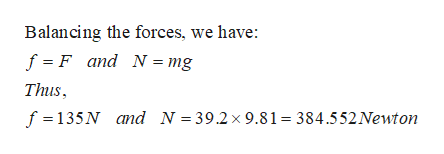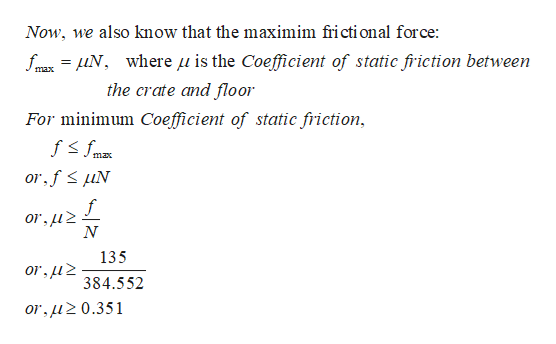# A woman exerts a horizontal force of 135 N on a box with a mass of 39.2 kg. (a)If the box doesn't move, what's the magnitude of the static friction force (in N)?  (b)What is the minimum possible value of the coefficient of static friction between the box and the floor? (Assume the box remains stationary.)

Question
3 views
A woman exerts a horizontal force of 135 N on a box with a mass of 39.2 kg.

(a)
If the box doesn't move, what's the magnitude of the static friction force (in N)?

(b)
What is the minimum possible value of the coefficient of static friction between the box and the floor? (Assume the box remains stationary.)

check_circle

Step 1

To find :

(a)The magnitude of the static friction force (in N)

(b)The minimum possible value of the coefficient of static friction between the box and the floorhelp_outlineImage TranscriptioncloseBalancing the forces, we have: f F and N = mg Thus f 135N and N = 39.2x 9.81 384.552Newton fullscreen
Step 2

Thus,

...help_outlineImage TranscriptioncloseNow, we also know that the maximim fricti onal force: fm Nwhere u is the Coefficient of static fric tion between max the crate and floor For minimum Coefficient of static friction max or fuN f or,u2 N 135 or,u 384.552 or,uz0.351 fullscreen

### Want to see the full answer?

See Solution

#### Want to see this answer and more?

Solutions are written by subject experts who are available 24/7. Questions are typically answered within 1 hour.*

See Solution
*Response times may vary by subject and question.
Tagged in

### Other